# 打印心形图案---程序猿才有的浪漫---入门级---C语言实现

2月前   阅读数 51 0

## 1.效果展示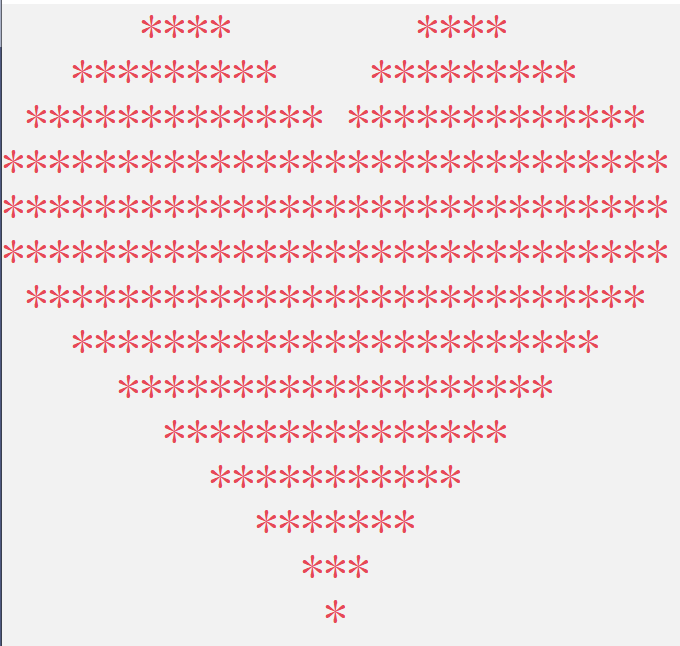## 2.打印思路

1.心形上方的两个梯形（3行）

2.心形中间的一个矩形（3行）

3.心形下方的一个倒三角形（8行）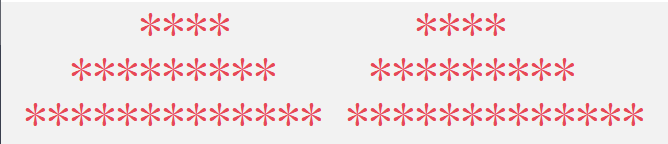为了照顾初学者，我们前三行的输出就暂且采用最笨的方法也是可以打印的。

``````    printf("      ****        ****\n");//打印第一行
printf("   *********    *********\n");//打印第二行
printf(" ************* *************\n");//打印第三行``````

第二部分，矩形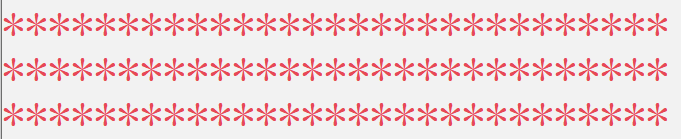打印三行，这三行的输出效果一样，每行29个 ' * '

``````    int i = 0;//定义变量
int j = 0;//定义变量
for (i = 0;i < 3;i++)//打印4-6行，一共3行，因此i小于3
{
for (j = 0;j < 29;j++)//限制每行输出*的个数
{
printf("*");//这三行只打印*号，无空格输出
}
printf("\n");//打印完一行需要进行换行
}``````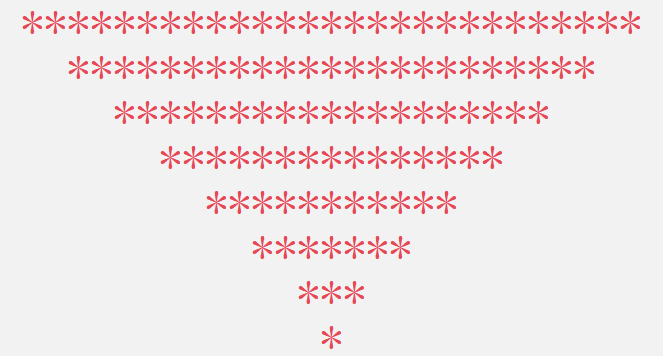这一部分需要一定的分析。第一行有一个空格，往下每行多两个空格，第一行有27个星号，往下每行少4个星号。

``````    for (i = 0;i < 7;i++) //打印7-13行，一共7行，因此i小于7
{
for (j = 0;j < 2 * (i + 1) - 1;j++)//这个for循环和下面的for是并列的
{
printf(" ");//打印空格
}
for (j = 0;j < 27 - i * 4;j++)//读者可以带入几个数找出条件
{
printf("*");//打印*
}
printf("\n");
}
for (i = 0;i < 14;i++)//打印最后一行的*
{
printf(" ");//打印空格
}
printf("*\n");//打印*``````

``````#include<stdio.h>//头文件
int main()//主函数入口
{
printf("      ****       ****\n");//打印第一行
printf("   *********   *********\n");//打印第二行
printf("************* *************\n");//打印第三行
int i, j;//定义变量
for (i = 0;i < 3;i++)//打印4-6行，一共3行，因此i小于3
{
for (j = 0;j < 29;j++)//限制每行输出*的个数
{
printf("*");//这三行只打印*号，无空格输出
}
printf("\n");//打印完一行需要进行换行
}
for (i = 0;i < 7;i++) //打印7-13行，一共7行，因此i小于7
{
for (j = 0;j < 2 * (i + 1) - 1;j++)//这个for循环和下面的for是并列的
{
printf(" ");//打印空格
}
for (j = 0;j < 27 - i * 4;j++)//读者可以带入几个数找出条件
{
printf("*");//打印*
}
printf("\n");
}
for (i = 0;i < 14;i++)//打印最后一行的*
{
printf(" ");//打印空格
}
printf("*\n");//打印*
return 0;
}``````

## 3.配色优化（修改控制台配色）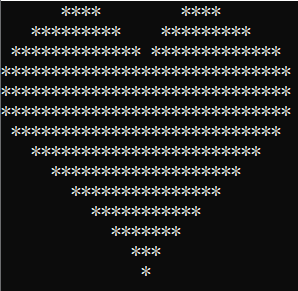我们可以通过以下方式进行自选配色。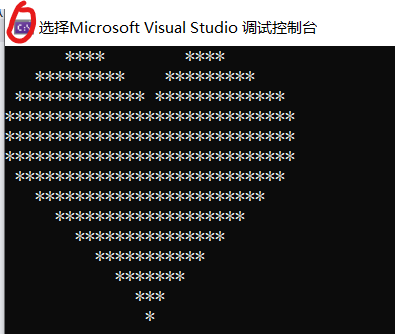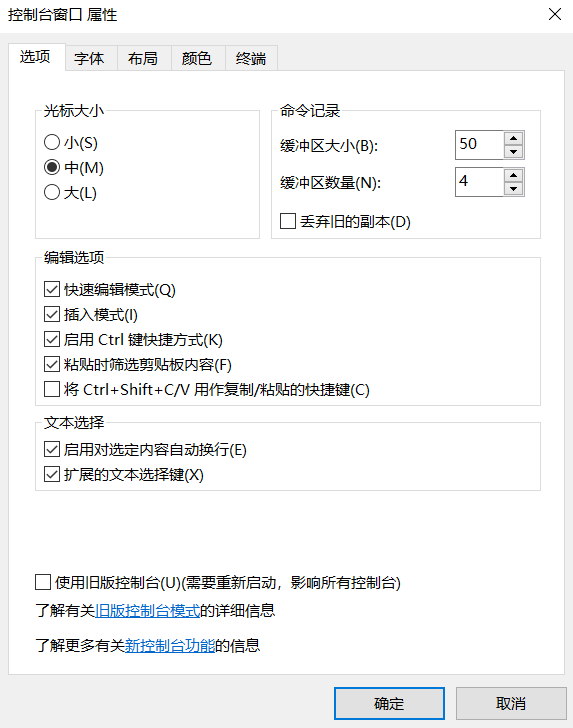以下是小作者选用的字体和配色所产生的效果：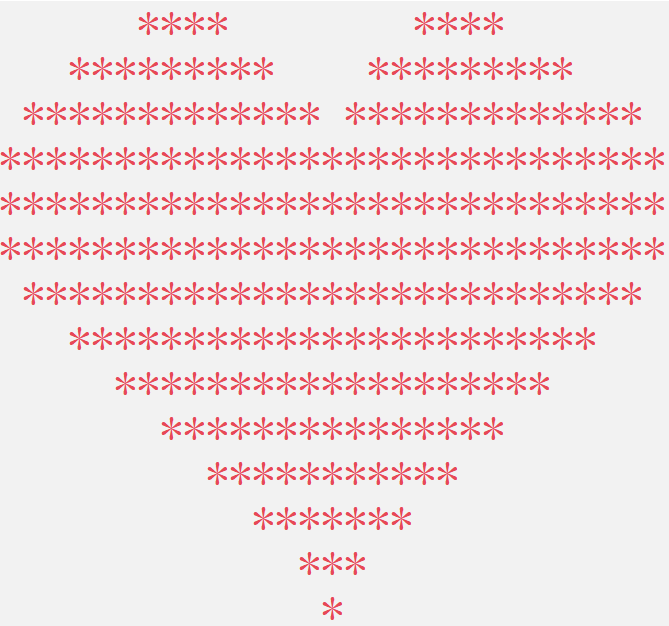文章到这里就结束了，给小作者一个赞，就是读者就是最大的爱😘

我有话说: